Courses

# Test: Ratio And Proportion- 3

## 15 Questions MCQ Test General Aptitude for GATE 2020 | Test: Ratio And Proportion- 3

Description
This mock test of Test: Ratio And Proportion- 3 for GATE helps you for every GATE entrance exam. This contains 15 Multiple Choice Questions for GATE Test: Ratio And Proportion- 3 (mcq) to study with solutions a complete question bank. The solved questions answers in this Test: Ratio And Proportion- 3 quiz give you a good mix of easy questions and tough questions. GATE students definitely take this Test: Ratio And Proportion- 3 exercise for a better result in the exam. You can find other Test: Ratio And Proportion- 3 extra questions, long questions & short questions for GATE on EduRev as well by searching above.
QUESTION: 1

### Which of the following fractions is at least twice as great as 11/50?

Solution:

First, let us rephrase the question. Since we need to find the fraction that is at least twice greater than 11/50, we are looking for a fraction that is equal to or greater than 22/50. Further, to facilitate our analysis, note that we can come up with an easy benchmark value for this fraction by doubling both the numerator and the denominator and thus expressing it as a percent: 22/50 = 44/100 = 44%. Thus, we can rephrase the question: “Which of the following is greater than or equal to 44%?”

Now, let’s analyze each of the fractions in the answer choices using benchmark values:
2/5: This fraction can be represented as 40%, which is less than 44%.
11/34: This value is slightly less than 11/33 or 1/3. Therefore, it is smaller than 44%.
43/99: Note that the fraction 43/99 is smaller than 44/100, since fractions get smaller if the same number (in this case integer 1) is subtracted from both the numerator and the denominator.
8/21: We know that 8/21 is a little less than 8/20 or 2/5. Thus, 8/21 is less than 44%.
9/20: Finally, note that by multiplying the numerator and the denominator by 5, we can represent this fraction as 45/100, thus concluding that this fraction is greater than 44%.

QUESTION: 2

### At the beginning of the year, the ratio of juniors to seniors in high school X was 3 to 4. During the year, 10 juniors and twice as many seniors transferred to another high school, while no new students joined high school X. If, at the end of the year, the ratio of juniors to seniors was 4 to 5, how many seniors were there in high school X at the beginning of the year?

Solution:

Let’s denote the number of juniors and seniors at the beginning of the year as j and s, respectively. At the beginning of the year, the ratio of juniors to seniors was 3 to 4:
j/s = 3/4. Therefore, j = 0.75s

At the end of the year, there were (j - 10) juniors and (s - 20) seniors. Additionally, we know that the ratio of juniors to seniors at the end of the year was 4 to 5. Therefore, we can create the following equation: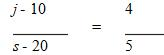Let’s solve this equation by substituting j = 0.75s: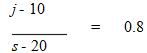(j - 10) = 0.8(s - 20)
(0.75s - 10) = 0.8s - 16
0.8s - 0.75s = 16 - 10
0.05s = 6
s = 120

Thus, there were 120 seniors at the beginning of the year.

QUESTION: 3

### 3/5 of a certain class left on a field trip. 1/3 of the students who stayed behind did not want to go on the field trip (all the others did want to go). When another vehicle was located, 1/2 of the students who did want to go on the field trip but had been left behind were able to join. What fraction of the class ended up going on the field trip?

Solution:

For a fraction question that makes no reference to specific values, it is best to assign a smart number as the "whole value" in the problem. In this case we'll use 30 since that is the least common denominator of all the fractions mentioned in the problem.

If there are 30 students in the class, 3/5 or 18, left for the field trip. This means that 12 students were left behind.

1/3 of the 12 students who stayed behind, or 4 students, didn't want to go on the field trip.

This means that 8 of the 12 who stayed behind did want to go on the field trip.

When the second vehicle was located, half of these 8 students or 4, were able to join the other 18 who had left already.

That means that 22 of the 30 students ended up going on the trip. 22/30 reduces to 11/15 so the correct answer is C.

QUESTION: 4

The ratio of boys to girls in Class A is 3 to 4. The ratio of boys to girls in Class B is 4 to 5. If the two classes were combined, the ratio of boys to girls in the combined class would be 17 to 22. If the number of boys in Class B is one less than the number of boys in Class A, and if the number of girls in Class B is two less than the number of girls in Class A, how many girls are in Class A?

Solution:

The ratio of boys to girls in Class A is 3 to 4. We can represent this as an equation: b/g = 3/4. We can isolate the boys:
4b = 3g
b = (3/4)g

Let's call the number of boys in Class B x, and the number of girls in Class B y

We know that the number of boys in Class B is one less than the number of boys in Class A. Therefore, x = b – 1. We also know that the number of girls in Class B is two less than the number of girls in Class A. Therefore, y = g – 2. We can substitute these in the combined class equation:
The combined class has a boy/girl ratio of 17 to 22: (b + x)/(g + y) = 17/22.
(b + b – 1)/(g + g – 2) = 17/22
(2b – 1)/(2g – 2) = 17/22

Cross-multiplying yields:
44b – 22 = 34g – 34
Since we know that b = (3/4)g, we can replace the b:
44(3/4)g – 22 = 34g – 34
33g – 22 = 34g – 34
12 = g
Alternatively, because the numbers in the ratios and the answer choices are so low, we can try some real numbers.  The ratio of boys to girls in Class A is 3:4, so here are some possible numbers of boys and girls in Class A:
B:G
3:4
6:8
9:12
The ratio of boys to girls in Class B is 4:5, so here are some possible numbers of boys and girls in Class A:
B:G
4:5
8:10
12:15
We were told that there is one more boy in Class A than Class B, and two more girls in Class A than Class B.  If we look at our possibilities above, we see that this information matches the case when we have 9 boys and 12 girls in Class A and 8 boys and 10 girls in Class B.  Further, we see we would have 9 + 8 = 17 boys and 12 + 10 = 22 girls in a combined class, so we have the correct 17:22 ratio for a combined class.  We know now there are 12 girls in Class A.

QUESTION: 5

John's front lawn is 1/3 the size of his back lawn. If John mows 1/2 of his front lawn and 2/3 of his back
lawn, what fraction of his lawn is left unmowed?

Solution:

We can solve this problem by choosing a smart number to represent the size of the back lawn.  In this case, we want to choose a number that is a multiple of 2 and 3 (the denominators of the fractions given in the problem).  This way, it will be easy to split the lawn into halves and thirds.  Let's assume the size of the back lawn is 6.

(size back lawn) = 6 units
(size front lawn) = (1/3)(size back lawn) = 2 units
(size total lawn) = (size back lawn) + (size front lawn) = 8 units

Now we can use these numbers to calculate how much of each lawn has been mowed:

Front lawn:  (1/2)(2) = 1 unit
Back lawn:  (2/3)(6) = 4 unit

So, in total, 5 units of lawn have been mowed.  This represents 5/8 of the total, meaning 3/8 of the lawn is left unmowed.

Alternatively, this problem can be solved using an algebraic approach.  Let's assume the size of the front lawn is x and size of the back lawn is y.  So, John has mowed (1/2)x and (2/3)y, for a total of (1/2)x + (2/3)y.  We also know that x = (1/3)y.  Substituting for x gives:

(1/2)x + (2/3)y
(1/2)(1/3)y + (2/3)y
(1/6)y + (2/3)y
(5/6)y = lawn mowed

The total lawn is the sum of the front and back, x + y.  Again, substituting for x gives (1/3)y + y = (4/3)y.  So, the fraction of the total lawn mowed is: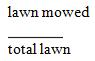= (5/6) × (3/4) = 15/24 = 5/8.
This leaves 3/8 unmowed.

QUESTION: 6

At Jefferson Elementary School, the number of teachers and students (kindergarten through sixth grade) totals 510. The ratio of students to teachers is 16 to 1. Kindergarten students make up 1/5 of the student population and fifth and sixth graders account for 1/3 of the remainder. Students in first and second grades account for 1/4 of all the students. If there are an equal number of students in the third and fourth grades, then the number of students in third grade is how many greater or fewer than the number of students in kindergarten?

Solution:

We know that the student to teacher ratio at the school is 16 to 1, and the total number of people is 510.  Therefore:
Number of students = (16/17)(510) = 480
Number of teachers = (1/17)(510) = 30

Kindergarten students make up 1/5 of the student population, so:
Number of kindergarten students = (1/5)(480) = 96

Fifth and sixth graders account for 1/3 of the remainder (after kindergarten students are subtracted from the total), therefore:
Number of 5th and 6th grade students = (1/3)(480 – 96) = (1/3)(384) = 128

Students in first and second grades account for 1/4 of all the students, so:
Number of 1st and 2nd grade students = (1/4)(480) = 120

So far, we have accounted for every grade but the 3rd and 4th grades, so they must consist of the students left over:
Number of 3rd and 4th grade students = Total students – students in other grades
Number of 3rd and 4th grade students = 480 – 96 – 128 – 120 = 136

If there are an equal number of students in the third and fourth grades, then:
Number of 3rd grade students = 136/2 = 68

The number of students in third grade is 68, which is fewer than 96, the number of students in kindergarten. The number of students in 3rd grade is thus 96 – 68 = 28 fewer than the number of kindergarten students.

QUESTION: 7

A certain galaxy is known to comprise approximately 4 x 1011 stars. Of every 50 million of these stars, one is larger in mass than our sun. Approximately how many stars in this galaxy are larger than the sun?

Solution:

50 million can be represented in scientific notation as 5 x 107. Restating this figure in scientific notation will enable us to simplify the division required to solve the problem. If one out of every 5 x 107 stars is larger than the sun, we must divide the total number of stars by this figure to find the solution: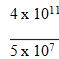= 4/5 x 10(11-7)

= 0.8 x 104

The final step is to move the decimal point of 0.8 four places to the right, with a result of 8,000.

QUESTION: 8

A lemonade stand sold only small and large cups of lemonade on Tuesday. 3/5 of the cups sold were small and the rest were large. If the large cups were sold for 7/6 as much as the small cups, what fraction of Tuesday's total revenue was from the sale of large cups?

Solution:

For a fraction word problem with no actual values for the total, it is best to plug numbers to solve.

Since 3/5 of the total cups sold were small and 2/5 were large, we can arbitrarily assign 5 as the number of cups sold.

Total cups sold = 5
Small cups sold = 3
Large cups sold = 2

Since the large cups were sold at 7/6 as much per cup as the small cups, we know:
Pricelarge = (7/6)Pricesmall
Let's assign a price of 6 cents per cup to the small cup.

Price of small cup = 6 cents
Price of large cup = 7 cents

Now we can calculate revenue per cup type:
Large cup sales = quantity × cost = 2 × 7 = 14 cents
Small cup sales = quantity × cost = 3 × 6 = 18 cents
Total sales = 32 cents

The fraction of total revenue from large cup sales = 14/32 = 7/16.

QUESTION: 9

Miguel is mixing up a salad dressing. Regardless of the number of servings, the recipe requires that 5/8 of the finished dressing mix be olive oil, 1/4 vinegar, and the remainder an even mixture of salt, pepper and sugar. If Miguel accidentally doubles the vinegar and forgets the sugar altogether, what proportion of the botched dressing will be olive oil?

Solution:

This problem can be solved most easily by picking smart numbers and assigning values to the portion of each ingredient in the dressing. A smart number in this case would be one that enables you to add and subtract ingredients without having to deal with fractions or decimals. In a fraction problem, the ‘smart number’ is typically based on the least common denominator among the given fractions.

The two fractions given, 5/8 and 1/4, have a least common denominator of 8. However, we must also consider the equal parts salt, pepper and sugar. Because 1/4 = 2/8, the total proportion of oil and vinegar combined is 5/8 + 2/8 = 7/8. The remaining 1/8 of the recipe is split three ways: 1/24 each of salt, pepper, and sugar. 24 is therefore our least common denominator, suggesting that we should regard the salad dressing as consisting of 24 units. Let’s call them cups for simplicity, but any unit of measure would do. If properly mixed, the dressing would consist of

5/8 × 24 = 15 cups of olive oil
1/4 × 24 = 6 cups of vinegar
1/24 × 24 = 1 cup of salt
1/24 × 24 = 1 cup of sugar
1/24 × 24 = 1 cup of pepper

Miguel accidentally doubled the vinegar and omitted the sugar. The composition of his bad salad dressing would therefore be

15 cups of olive oil
12 cups of vinegar
1 cup of salt
1 cup of pepper

The total number of cups in the bad dressing equals 29. Olive oil comprises 15/29 of the final mix.

QUESTION: 10

Harold and Millicent are getting married and need to combine their already-full libraries. If Harold, who has 1/2 as many books as Millicent, brings 1/3 of his books to their new home, then Millicent will have enough room to bring 1/2 of her books to their new home. What fraction of Millicent's old library capacity is the new home's library capacity?

Solution:

This problem never tells us how many books there are in any of the libraries. We can, therefore, pick numbers to represent the quantities in this problem. It is a good idea to pick Smart Numbers, i.e. numbers that are multiples of the common denominator of the fractions given in the problem.
In this problem, Harold brings 1/3 of his books while Millicent brings 1/2.  The denominators, 2 and 3, multiply to 6, so let's set Harold's library capacity to 6 books.  The problem tells us Millicent has twice as many books, so her library capacity is 12 books.  We use these numbers to calculate the size of the new home's library capacity.  1/3 of Harold's 6-book library equals 2 books.  1/2 of Millicent's 12-book library equals 6 books.  Together, they bring a combined 8 books to fill their new library.
The fraction we are asked for, (new home's library capacity) / (Millicent's old library capacity), therefore, is 8/12, which simplifies to 2/3.

QUESTION: 11

In a certain pet shop, the ratio of dogs to cats to bunnies in stock is 3 : 5 : 7. If the shop carries 48 cats and bunnies total in stock, how many dogs are there?

Solution:

The ratio of dogs to cats to bunnies (Dogs: Cats: Bunnies) can be expressed as 3x: 5x : 7x. Here, x represents an "unknown multiplier."  In order to solve the problem, we must determine the value of the unknown multiplier.

Cats + Bunnies = 48
5x + 7x = 48
12x = 48
x = 4

Now that we know that the value of x (the unknown multiplier) is 4, we can determine the number of dogs.

Dogs = 3x = 3(4) = 12

QUESTION: 12

A foreign language club at Washington Middle School consists of n students, 2/5 of whom are boys. All of the students in the club study exactly one foreign language. 1/3 of the girls in the club study Spanish and 5/6 of the remaining girls study French. If the rest of the girls in the club study German, how many girls in the club, in terms of n, study German?

Solution:

Boys = 2n/5, girls = 3n/5
3n
Girls studying Spanish =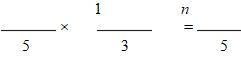3n
Girls not studying Spanish =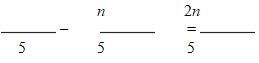2n
Girls studying French =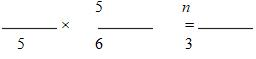Girls studying German = (all girls) – (girls studying Spanish) – (girls studying French)

3n
Girls studying German =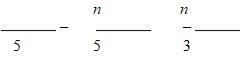2n
Girls studying German =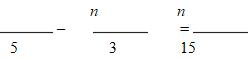The correct answer is E

QUESTION: 13

A certain ball team has an equal number of right- and left-handed players. On a certain day, two-thirds of the players were absent from practice. Of the players at practice that day, one-third were left handed. What is the ratio of the number of right-handed players who were not at practice that day to the number of lefthanded players who were not at practice?

Solution:

Since the problem deals with fractions, it would be best to pick a smart number to represent the number of ball players. The question involves thirds, so the number we pick should be divisible by 3. Let's say that we have 9 right-handed players and 9 left-handed players (remember, the question states that there are equal numbers of righties and lefties).
Two-thirds of the players are absent from practice, so that is (2/3)(18) = 12. This leaves 6 players at practice. Of these 6 players, one-third were left-handed. This yields (1/3)(6) = 2 left-handed players at practice and 9 – 2 = 7 left-handed players NOT at practice. Since 2 of the 6 players at practice are lefties, 6 – 2 = 4 players at practice must be righties, leaving 9 – 4 = 5 righties NOT at practice.
The question asks us for the ratio of the number of righties not at practice to the number of lefties not at practice. This must be 5 : 7 or 5/7.

QUESTION: 14

Bag A contains red, white and blue marbles such that the red to white marble ratio is 1:3 and the white to blue marble ratio is 2:3. Bag B contains red and white marbles in the ratio of 1:4. Together, the two bags contain 30 white marbles. How many red marbles could be in bag A?

Solution:

We are told that bag B contains red and white marbles in the ration 1:4. This implies that WB, the number of white marbles in bag B, must be a multiple of 4.

What can we say about WA, the number of white marbles in bag A? We are given two ratios involving the white marbles in bag A. The fact that the ratio of red to white marbles in bag A is 1:3 implies that WA must be a multiple of 3. The fact that the ratio of white to blue marbles in bag A is 2:3 implies that WA must be a multiple of 2. Since WA is both a multiple of 2 and a multiple of 3, it must be a multiple of 6.
We are told that WA + WB = 30. We have already figured out that WA must be a multiple of 6 and that WB must be a multiple of 4. So all we need to do now is to test each candidate value of WA (i.e. 6, 12, 18, and 24) to see whether, when plugged into WA + WB = 30, it yields a value for WB that is a multiple of 4. It turns out that WA = 6 and WA = 18 are the only values that meet this criterion.
Recall that the ratio of red to white marbles in bag A is 1:3. If there are 6 white marbles in bag A, there are 2 red marbles. If there are 18 white marbles in bag A, there are 6 red marbles. Thus, the number of red marbles in bag A is either 2 or 6. Only one answer choice matches either of these numbers.

QUESTION: 15

The ratio by weight, measured in pounds, of books to clothes to electronics in Jorge's suitcase initially stands at 8 to 5 to 3. Jorge then removes 4 pounds of clothing from his suitcase, thereby doubling the ratio of books to clothes. Approximately how much do the electronics in the suitcase weigh, to the nearest pound?

Solution:

Initially the ratio of B: C: E can be written as 8x: 5x: 3x.  (Recall that ratios always employ a common multiplier to calculate the actual numbers.)
After removing 4 pounds of clothing, the ratio of books to clothes is doubled. To double a ratio, we double just the first number; in this case, doubling 8 to 5 yields a new ratio of 16 to 5. This can be expressed as follows: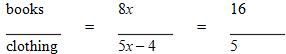Cross multiply to solve for x:

40x = 80x – 64
40x = 64
x = 8/5
The question asks for the approximate weight of the electronics in the suitcase. Since there are 3x pounds of electronics there are 3 × (8/5) = 24/5 or approximately 5 pounds of electronics in the suitcase.